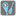• # Digits Multiplication

## Question related to missionDigits Multiplication

I wrote my code us carried out it, but an error [Assertion Error] occurred. I see this error for the first time, and there is no hint to change my code. Of course I searched on the web, but I couldn't find any useful article to solve this problem.

Here is my code...

```def checkio(number: int) -> int:
number_str = str(number)
number_list = list(number_str)
exclude_strlist = [i for i in number_list if not i == 0]
exclude_intlist = [int(s) for s in exclude_strlist]
a = 1
for i in range(len(exclude_intlist)):
a = a * exclude_intlist[i]
return a
```